## Снимки экрана

•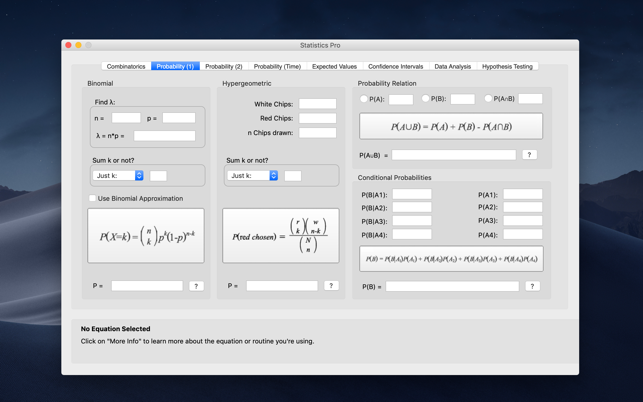•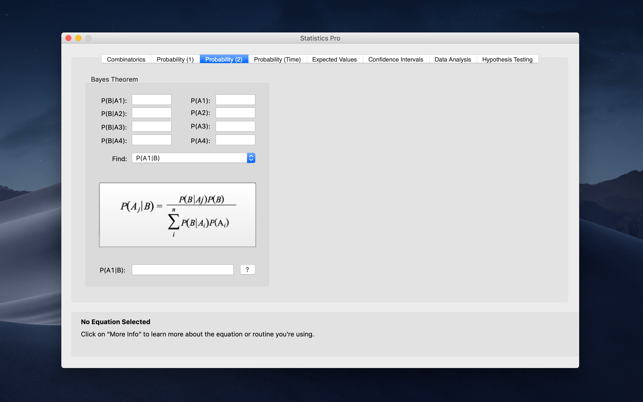•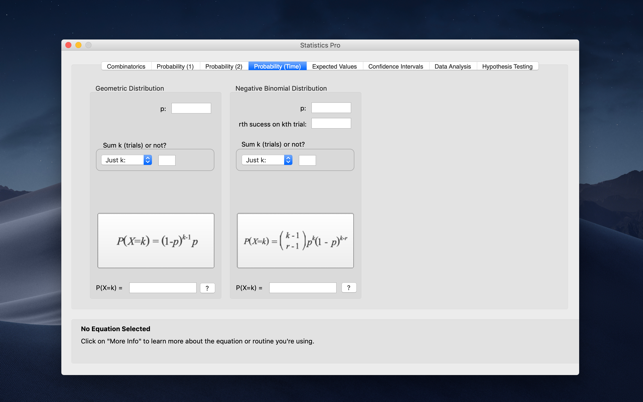•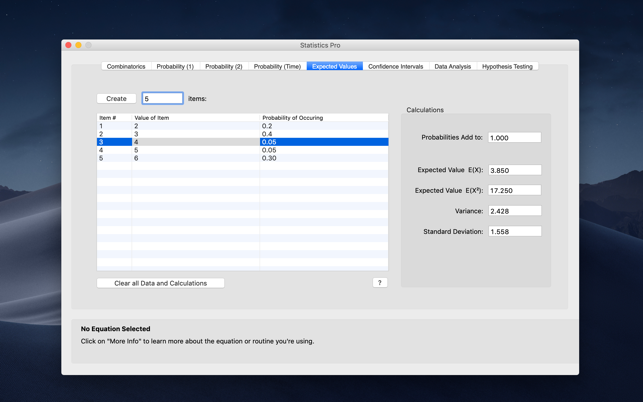•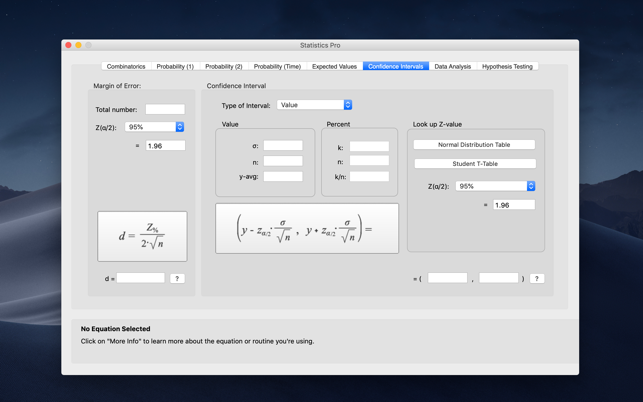•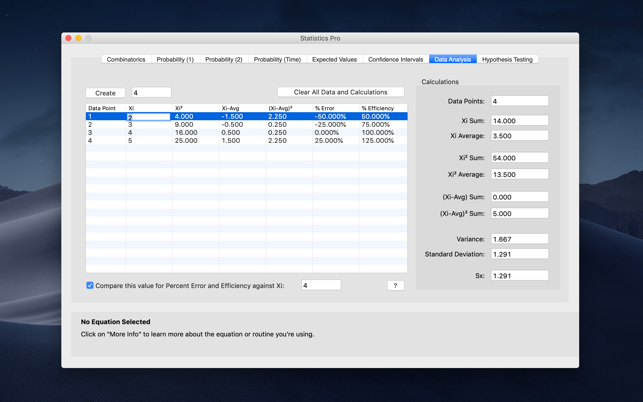•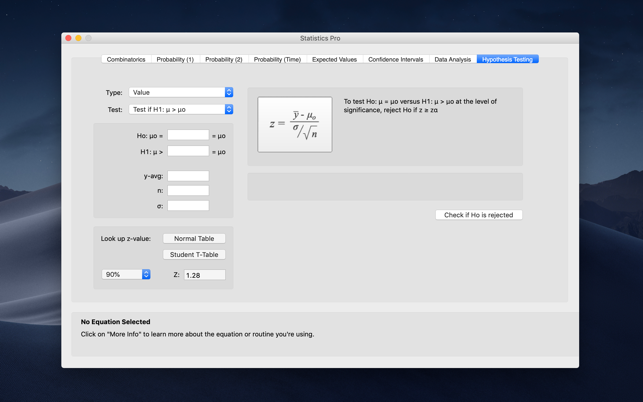•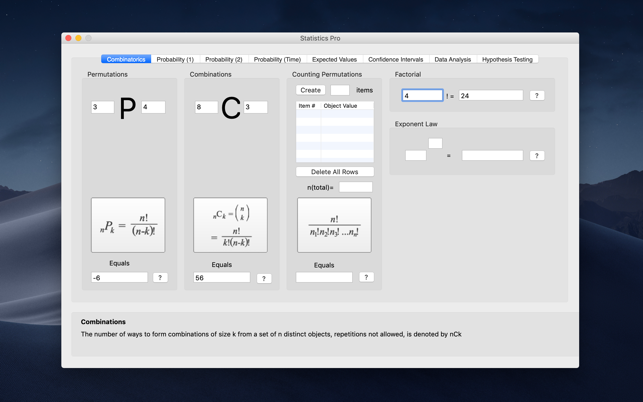## Описание

Statistics is a great class, but the nature of the calculations in homework, quizes and tests can be tedious and time-consuming. You can fully understand the formula you're working with and the calculation you're doing, but one slight miscalculation will affect the entire formula and produce a wrong answer!

Introducing Statistics Pro -- the program specifically designed to quickly and accurately calculate the most common formulas found in Statistics, Business Statistics, and Discrete Mathematics/Structures classes.

Statistics Pro does more than simply give you the correct answer! It helps to ensure your understanding of the formulas and concepts in your homework and tests by showing, and explaining, the actual formula you're using, all with a simple click of the handy "?" button available for every formula.

Easy-to-use and fast!
All equations and methods are just a click away, providing the fast calculation you'll definitely appreciate over hours of button mashing on a calculator. Most of them are automatically calculated as you enter your data, allowing you to quickly see how changing one variable affects the entire result. Equations that take 10-15 minutes on a regular calculator take just seconds with Statistics Pro!

Formula Guide
The full version of Statistics Pro even provides our PDF guide for Statistics formulas, teaching you -- in simple terms and with almost 20 pages of visual examples -- every formula found in the program, and demonstrates how easy and fast it is to use the program to get the answers you need.

Statistics Pro contains these exciting features:
Combinatorics - Calculate permutations, combinations, counting permutations, factorials and more.

Probability - Binomial probability, hypergeometric probability, conditional probability, probability relation and Bayes Thereom.

Probability with time intervals - Calculate probability with time intervals with the geometric and negative binomial distribution.

Expected Values - Use the expected values method to quickly find the expected value, variance and standard deviation.

Confidence Intervals - Confidently and quickly calculate confidence intervals with a given confidence level.

Data Analysis - This computes tedious sums, squares of differences, and more to ultimately find the standard deviation and more.

Hypothesis Testing -- enter a few values and with the click of a mouse, know whether your null hypothesis is accepted.

## Что нового

Версия 1.4

Recompiled in 64-bit

## Информация

Провайдер
Jake Mavity
Размер
4.8 МБ
Категория
Образование
Совместимость

OS X 10.10 и новее, 64-разрядный процессор

Языки

английский

Возраст
Возраст: 4+
Copyright
©2008-2020 Praeter Software
Цена
149 р.

## Поддерживается

•